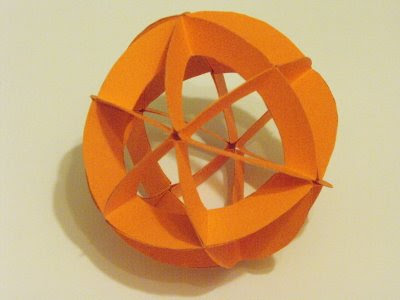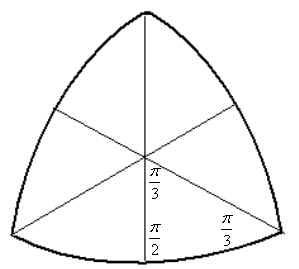## Wednesday, April 8, 2009

### Spherical TetrahedronImagine a tetrahedron centered inside a sphere. If you were to project the edges of the tetrahedron out from the center so that they touched the surface of the sphere, the edges would cut the sphere in arcs that lie on the sphere's 'great circles' (the largest possible circles drawn on the surface of the sphere - circles whose radii are the same as the radius of the sphere).

The image at the top of this post shows a model of a sphere with the six great circles formed by the projected edges of a tetrahedron sitting at its center.

The model, described on pages 4 and 5 of Magnus Wennigers' Polyhedron Models, is easy to construct using the template shown below. Printing copies of the template onto card-stock and gluing them together works well. This pdf file has 10 arcs on a single page for printing (you will need 24 arcs in total).Each arc is folded and glued to form a spherical triangle, 24 of the spherical triangles glued together will form the sphere.

The diagram below (based on Fig.2, p.5, in Wenniger) shows how to assemble 6 of the triangles into one 'face' of the spherical tetrahedron. Four of the faces can be assembled into the 'spherical tetrahedron.' When assembling, you should make sure that you align the triangles so that they form great circles.Spherical triangles, as you can tell from the diagram, do not follow the rule of having their interior angles sum to pi (180 degrees), as plane triangles do. See wikipedia and mathworld for more on the relations that govern spherical triangles.

The arc is divided into angles of 54deg&44min, 70deg&32min, 54deg&44min, as described by Wenniger. The template was created in GSP.